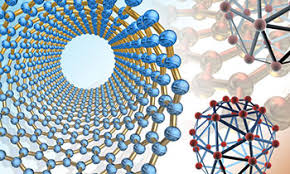## Mechanical & Materials Engineering, Department of## Mechanical & Materials Engineering Faculty Publications

8-1992

#### Comments

Published in Journal of Applied Physics 72:4 (August 15, 1992), pp. 1362-1373. Copyright 1992 American Institute of Physics. Used by permission.

#### Abstract

Exact expressions are presented for the deflection of a laser beam passing parallel to and above the surface of a sample heated by a periodically modulated axisymmetric laser beam. The sample may consist of any number of planar films on a thick substrate. These exact expressions are derived from a local Green’s function treatment of the heat conduction equation, and contain an exact analytical treatment of the absorption of energy in the multilayered system from the heating laser. The method is based on calculation of the normal component of the heat fluxes across the layer boundaries, from which either the beam deflections or the temperature anywhere in space can be easily found. A central part of the calculation is a tridiagonal matrix equation for the N + 1 normal boundary fluxes, where N equals the number of films in the sample, with the beam deflections given as simple functions of the normal heat flux through the top surface of the sample. Even though any layer or layers in the sample (including the substrate) can be optically absorbing, the final results are remarkably simple both in form and ease of calculation, even for large numbers of layers. In the case of an infinitesimal probe beam, the beam deflections are given by an expression involving a single numerical integration which can be eliminated for data analysis by Fourier transforming the experimental data. A general expression for the measured signals for the case of four-quadrant detection is also presented and compared to previous calculations of detector response for finite probe beams.

COinS